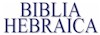Lesson I Lesson II Lesson III Lesson IV Lesson V Lesson VI Lesson VII Lesson VIII Lesson IX Lesson X Lesson XI Lesson XII Lesson XIII Lesson XIV Lesson XV Lesson XVI Lesson XVII Lesson XVIII Lesson XIX Lesson XX Lesson XXI Lesson XXII Lesson XXIII Lesson XXIV Lesson XXV Lesson XXVI Lesson XXVII Lesson XXVIII Lesson XXIX Lesson XXX Lesson XXXI Notes Vocabulary Quiz Exercises

## LESSON I

• The Hebrew Alphabet - is made up of 22 consonants (counting \$ and & as a variation of one consonant)
• Hebrew is written and pronounced from right to left.  Transliteration is written and pronounced from left to right.
• The dagesh lene (•) hardens the sound of the consonant. There are six consonants that this occurs in.
• They are referred to as the BeGaD KeFaT letters: TPKDGB
• There are five consonants that are referred to as gutturals because they are made with sounds that come from the throat.
• They are )hx(r
• There are five consonants that are referred to as sibilants because they contain the "s" sound.
• They are zsc&\$
• Please note the transliteration of each letter and the pronunciation.

 NAME FORM FINAL FORM PRONUNCIATION aleph ) silent bet b v as in vine bet with dagesh B b as in boy gimel g g as in go gimel with dagesh G g as in go dalet d d as in dare dalet with dagesh D d as in dare he h h as in his vav w v as in vine zayin z z as in zeal het x ch as in Bach tet + t as in tall yod y y as in yes kaf with dagesh K k as in keep kaf k \ ch as in Bach lamed l l as in let mem m { m as in met nun n } n as in net samek s s as in set ayin ( silent pe p v f as in fat pe with dagesh P p as in pet sade c j ts as in nets qof q k as in keep resh r r as in rule sin & s as in sun shin \$ sh as shine tav t t as in tall tav with a dagesh T t as in tall